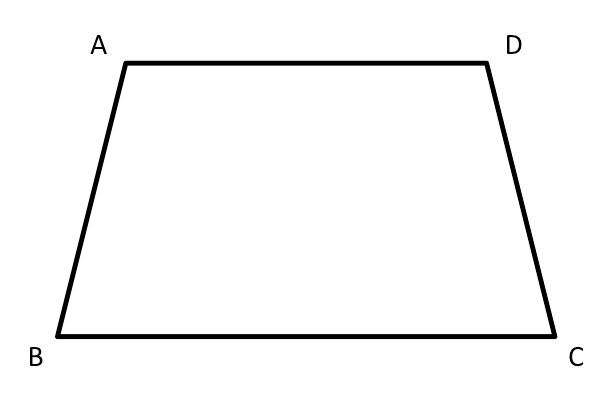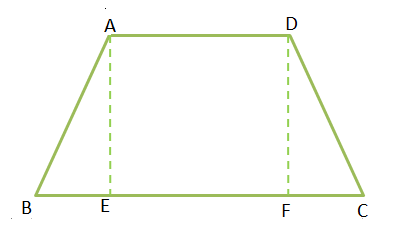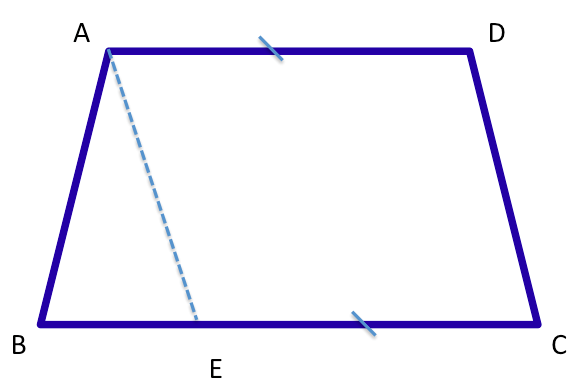D

#### Isosceles trapezoid

86 viewed last edited 4 years agoAnthony Schwartz
2How do I prove that the adjacent angles are congruent? That is in the isosceles trapezoid ABCD where AB \cong CD and AD \| BC how do I show that \angle{ABC} \cong \angle{BCD} and \angle{DAB} \cong \angle{CDA} ?Krishna
2Given that: ABCD is a isosceles trapezoid AB \cong CD and AD \| BC To show: \angle{ABC} \cong \angle{BCD} \angle{DAB} \cong \angle{CDA} Draw two perpendiculars to the base AE and DF AE=DF since they are altitudes of the same trapezoid AB=CD given in the question. Two right triangles are congruent if their hypotenuses are congruent and a corresponding leg is congruent. BE=CF Triangle ABE and Triangle DFC are congruent. So their angles are also equal. \angle{ABC} \cong \angle{BCD} (angles BCD=FCD)Mahesh Godavarti
1Draw a new line segment AE such that AD \cong EC as shown in the figure. Then since, AD \cong EC and AD \| EC , \square AECD is a parallelogram. Therefore, AE \cong CD and \triangle ABE is an isosceles triangle. After that, essentially, we have: 1. \angle EAD \cong \angle BCD Reason: \square AECD is a parallelogram. 2. \angle EAD \cong \angle AEB Reason: Alternate interior angles and AD \| BC 3. \angle AEB \cong \angle ABC Reason: In \triangle ABE , AB \cong AE . 4. \angle ABC \cong \angle BCD Reason: follows from Steps 1, 2 and 3. Similarly, we can prove for the other set of angles.